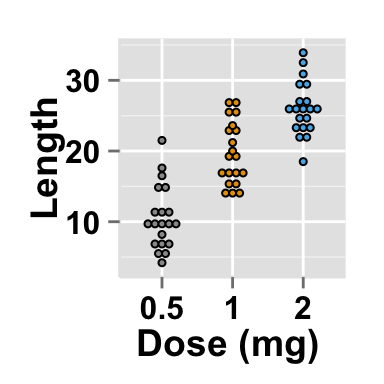# What is ggplot2 ?

ggplot2 is a powerful R package, implemented by Hadley Wickham, for producing nice graphs. The gg in ggplot2 means Grammar of Graphics, a graphic concept which describes plots by using a “grammar”. Plot can be divided in different fundamental parts : Plot <- data + Aesthetics + Geometry.

data are a data.frame Aesthetics is used to specify x and y variables. It can also be used to control the color, the size or the shape of a point, the height of a bar, etc….. Geometry corresponds to the type of graphs (histogram, box plot, line plot, density plot, dot plot, ….)

Read more —-> ggplot2 online documentation

# Introduction to easyGgplot2 package

“ggplot2 is too complicated: it is hard for me to improve, and hard for new developers to understand.” — Hadley Wickham 2011 (http://vita.had.co.nz/papers/future-ig.pdf)

The idea behind ggplot2 is seductively simple but the detail is, yes, difficult. To customize a plot, the syntax is sometimes a tiny bit opaque and this raises the level of difficulty.

For these reasons, I created the easyGgplot2 package (which depends on ggplot2) to make and customize quickly plots including box plot, dot plot, strip chart, violin plot, histogram, density plot, scatter plot, bar plot, line plot, etc, ….

In this chapter, you will find many tutorials on ggplot2 plotting systems and on how to personalize quickly graphical parameters including title, axis labels, legend, background and colors.

The syntax used to call easyGgplot2 functions is simple and it’s a kind of an easy interface between users and ggplot2 package. An example to illustrate the difference between the syntax of ggplot2 and easyGgplot2 is shown hereafter.

# Install and load easyGgplot2 package

To install the package, use the following R code from an R console (R >=3.1.0) :

``````install.packages("devtools")
library(devtools)
install_github("easyGgplot2", "kassambara")``````

Load the package using the R code:

``library(easyGgplot2)``

# Syntax of easyGgplot2 and ggplot2 packages

In the following R code, a box plot is drawn using either ggplot2 or easyGgplot2.

ggplot2 syntax :

``````ggplot(data=ToothGrowth, aes(x=dose, y=len, fill=dose))+
geom_boxplot()+
# Customize main title, x and y axis labels
ggtitle("Plot of length \n by dose") + xlab("Dose (mg)")+
theme(
# customize main title
plot.title = element_text( size=14, face="bold.italic",
colour="red", lineheight=1.0),
# customize x and y axis labels
axis.title.x=element_text(size=14, face="bold",
colour="blue", hjust=0.4),
axis.title.y=element_text(size=14, face="bold",
colour="blue", vjust=0.4, angle=90))+
#change group colors
scale_fill_manual(values=c('#999999','#E69F00','#56B4E9'))``````

easyGgplot2 syntax :

``````# Change main title and axis titles
# Customize title styles. Possible values for the font style :
# 'plain', 'italic', 'bold', 'bold.italic'.
ggplot2.boxplot(data=ToothGrowth, xName='dose',yName='len',
groupName="dose",
groupColors=c('#999999','#E69F00','#56B4E9'),
xtitle="Dose (mg)", ytitle="Length",
mainTitle="Plot of length \n by dose",
mainTitleFont=c(14,"bold.italic", "red"),
xtitleFont=c(14,"bold", "blue"),
ytitleFont=c(14,"bold", "blue"))``````Note that the outputs of the two packages are the same, and easyGgplot2 is just a wrapper for ggplot2 package

# Data visualizations

Click on the following articles to visualize your data using easyGgplot2 R package.

# ggplot2.boxplotggplot2.boxplot is an easy to use function from easyGgplot2 package to make and customize quickly a box plot using R software and ggplot2 plotting methods.

# ggplot2.dotplotggplot2.dotplot is an easy to use function from easyGgplot2 package to make and customize quickly a dot plot using R software and ggplot2 plotting methods.

# ggplot2.violinplotggplot2.violinplot is an easy to use function from easyGgplot2 package to make and customize quickly a violin plot using R software and ggplot2 plotting methods.

# ggplot2.stripchartggplot2.stripchart is an easy to use function from easyGgplot2 package to make and customize one dimensional scatter plots (or dot plots) using R software and ggplot2 package.

# ggplot2.histogramggplot2.histogram is an easy to use function from easyGgplot2 package to make and customize quickly histogram using R software and ggplot2 plotting method.

# ggplot2.densityggplot2.density is an easy to use function from easyGgplot2 package to make and customize quickly density curve using R software and ggplot2 package.

# ggplot2.customizeggplot2.customize is an easy to use function from easyGgplot2 package to customize plots (e.g : box and whisker plot, histogram, density plot, etc, …) generated with R easyGgplot2 or ggplot2 package.

# ggplot2.scatterplotggplot2.scatterplot is an easy to use function from easyGgplot2 package to make and customize quickly scatter plots using R software and ggplot2 package.

# ggplot2.multiplotggplot2.multiplot is a function, from easyGgplot2 package, to put multiple graphs on the same page.

# ggplot2.lineplotggplot2.lineplot is a function, from easyGgplot2 package, to easily draw and customize line plots.

# ggplot2.barplotggplot2.barplot is a function, from easyGgplot2 package, to easily draw and customize bar graphs using R software and ggplot2 plotting methods.

# Easy ggplot2 ebook

Note that an eBook is available on easyGgplot2 package here.

September 2014 : First edition.

Licence : This document is under creative commons licence (http://creativecommons.org/licenses/by-nc-sa/3.0/).

# Infos

This analysis was performed using R (ver. 3.1.0), easyGgplot2 (ver 1.0.0) and ggplot2 (ver 1.0.0).